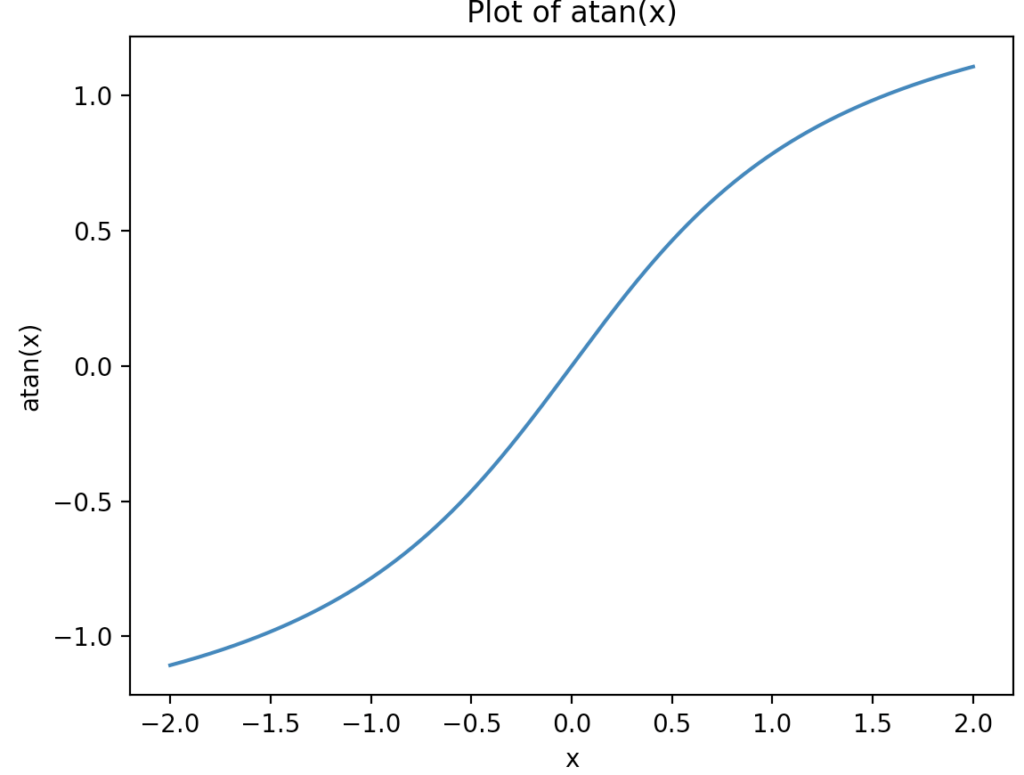# Python math.atan() Method

Python math.atan() method returns the arctangent of a number as a value.” The value passed to the atan() method should be between -PI/2 and PI/2 radians.

## Syntax

``math.atan(var)``

## Parameters

var: This parameter is the value passed to atan(). Any other data type is not acceptable. If any other data type is passed as a parameter, it throws a type error.

## Return Value

It returns the arc tan value of the number in the float datatype.

## Example 1: How to Use math.atan() Method

``````import math

var = 0.36
print(math.atan(var))``````

Output

``0.34555558058171215``

## Example 2: Passing positive and negative values to atan() method

``````import math

a1 = 0.36
b1 = 1
c1 = -1
d1 = -0.36

print("Value for parameter ", a1, " is ", math.atan(a1))
print("Value for parameter ", b1, " is ", math.atan(b1))
print("Value for parameter ", c1, " is ", math.atan(c1))
print("Value for parameter ", d1, " is ", math.atan(d1))``````

Output

``````Value for parameter 0.36 is 0.3455555805817121
Value for parameter 1 is 0.7853981633974483
Value for parameter -1 is -0.7853981633974483
Value for parameter -0.36 is -0.3455555805817121``````

## Example 3: Plotting the math.atan() method

``````import matplotlib.pyplot as plt
import math

# Create a list of x values from -2 to 2
x = [i * 0.01 for i in range(-200, 201)]

# Calculate the corresponding y values
y = [math.atan(i) for i in x]

# Create a new figure
plt.figure()

# Plot x against y
plt.plot(x, y)

# Set the title
plt.title('Plot of atan(x)')

# Set the x and y axis labels
plt.xlabel('x')
plt.ylabel('atan(x)')

# Display the plot
plt.show()``````

OutputThat’s it.

This site uses Akismet to reduce spam. Learn how your comment data is processed.